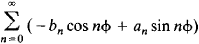# Conjugate Functions

The following article is from The Great Soviet Encyclopedia (1979). It might be outdated or ideologically biased.

## Conjugate Functions

Two functions u(x, y) and v(x, y) of the two variables x and y are said to be conjugate if in some region D they satisfy the Cauchy-Riemann equations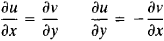Under certain conditions—for example, if u and v have continuous first-order partial derivatives— u and v are the real and imaginary parts, respectively, of some analytic function f(x + iy). They satisfy Laplace’s equation in D: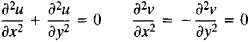That is, they are harmonic functions. If a harmonic function, such as u(x, y), is specified in a simply connected region D, the conjugate harmonic function v(x, y) and, consequently, the analytic function f(x + iy) are thereby uniquely defined (to within a constant term).

As an example, let us consider the function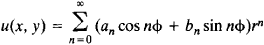where ø = arg(x + iy). If this function is harmonic within some circle ǀx + iyǀ = r < R, then its conjugate function is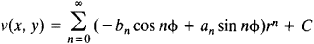and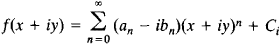The values of the conjugate functions on the circle r = 1 are periodic functions of ø and can be expanded in the conjugate trigonometric series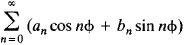and